# Colleges with the highest SAT scores in Minnesota

Top 10 colleges in Minnesota with the highest SAT scores
Looking for the colleges with the highest SAT scores in Minnesota? Well you're in luck! We've compiled a national college database and have created a list of the top 10 universities with the highest SAT scores in Minnesota. These are the schools whose applicants had the highest average SAT scores in Minnesota. And, since these tests are meant to determine academic prowess, they are arguably the schools with the most academically proficient students. You could even say these are the best colleges in Minnesota. We also include each college's ACT scores and acceptance rate so that you can see where you would have the easiest or hardest time getting in. Read on to find out more.

## Carleton College SAT scores

The average SAT score for Carleton College is 1450.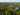The average SAT score of 1450 breaks down into:

• SAT math: 740

The average ACT score for Carleton College is 33 and their acceptance rate is 19%.

## Macalester College SAT scores

The average SAT score for Macalester College is 1411.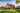The average SAT score of 1411 breaks down into:

• SAT math: 720

The average ACT score for Macalester College is 30 and their acceptance rate is 41.2%.

## University of Minnesota SAT scores

The average SAT score for University of Minnesota is 1352.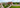The average SAT score of 1352 breaks down into:

• SAT math: 703

The average ACT score for University of Minnesota is 28 and their acceptance rate is 56.7%.

## Saint Olaf College SAT scores

The average SAT score for Saint Olaf College is 1300.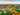The average SAT score of 1300 breaks down into:

• SAT math: 650

The average ACT score for Saint Olaf College is 29 and their acceptance rate is 49.9%.

## University of Saint Thomas SAT scores

The average SAT score for University of Saint Thomas is 1265.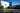The average SAT score of 1265 breaks down into:

• SAT math: 640

The average ACT score for University of Saint Thomas is 26 and their acceptance rate is 81.9%.

## Gustavus Adolphus College SAT scores

The average SAT score for Gustavus Adolphus College is 1260.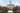The average SAT score of 1260 breaks down into:

• SAT math: 620

The average ACT score for Gustavus Adolphus College is 27 and their acceptance rate is 67.8%.

## Concordia College SAT scores

The average SAT score for Concordia College is 1200.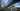The average SAT score of 1200 breaks down into:

• SAT math: 580

The average ACT score for Concordia College is 26 and their acceptance rate is 65.1%.

## College of Saint Benedict, Saint John's University SAT scores

The average SAT score for College of Saint Benedict, Saint John's University is 1170.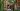The average SAT score of 1170 breaks down into:

• SAT math: 590

The average ACT score for College of Saint Benedict, Saint John's University is 25 and their acceptance rate is 80%.

## Hamline University SAT scores

The average SAT score for Hamline University is 1170.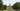The average SAT score of 1170 breaks down into:

• SAT math: 570

The average ACT score for Hamline University is 23 and their acceptance rate is 67.1%.

## University of Northwestern Saint Paul SAT scores

The average SAT score for University of Northwestern Saint Paul is 1163.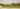The average SAT score of 1163 breaks down into:

• SAT math: 570